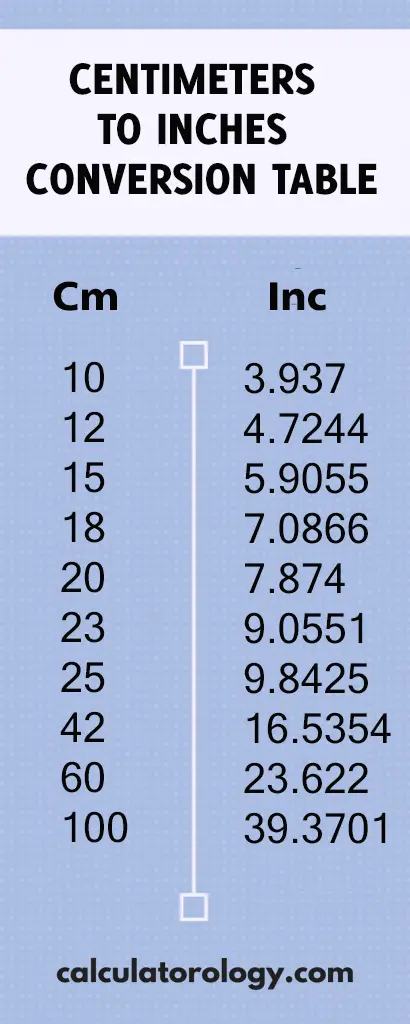код 12 cm to inches - Calculatorology

## 4.7244094488 inches

### 12 cm to inches

The Centimeters to Inches conversion calculator is used to convert centimeters (cm) to inches (in). To convert 12 centimeters to inches, it is important to determine the number of inches that are equivalent to one centimeter.

#### How many inches in one centimeter?

1 centimeter = 0.3937007874 Inch

This means that there are 0.3937007874 inches in one centimeter. It can also be converted into decimals and shown as;

1 cm = (1/2.54) = 0.3937007874 inches.

D (“) = D (cm)/2.54

Therefore, to determine the distance in inches, you will divide the distance in centimeters by 2.54.## 12 cm to Inches Conversion

 cm inches

#### How to convert 12cm to inches

When using the converter, the first procedure is to enter the value 12 in the blank text field and then click the ‘Convert’ button to initiate the conversion.  It gives you quick and accurate results based on what you enter in the blank text field.

Since 1 centimeter is equivalent to 0.3937007874 inches, how many inches are there in 12 centimeters?

If 1 cm = 0.3937007874 inches,

12cm =? Inches

= (12 x 0.3937007874) inches

=4.7244094488inches.

This implies that there are 4.7244094488inches in 12 centimeters.

The conversion calculator can still be used to perform more calculations using different values. The ‘Reset’ button is used to erase all information of the previous calculations to create a new platform for other calculations. The centimeter to inches conversion calculator can only execute a single conversion at a time.

The Centimeter to Inches conversion table can be used to determine the number of inches in 12 centimeters. It gives the conversion results of centimeters to inches based on a range of 0.01cm to 12cm.

##### Definitions

A centimeter is a length unit in the metric system abbreviated as "cm". One centimeter is equal to 0.3937007874 inches.

It is a US customary and imperial measurement system that is used widely and is abbreviated as (in) or using the symbol (“) - double prime.